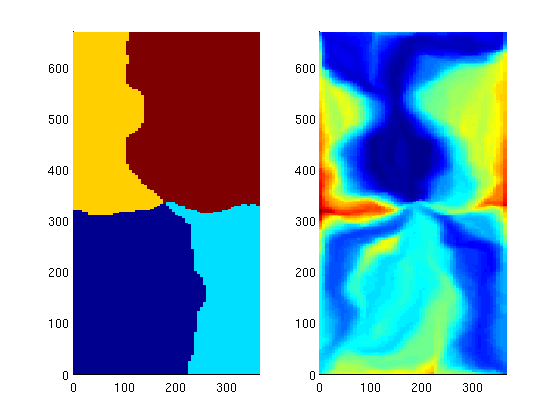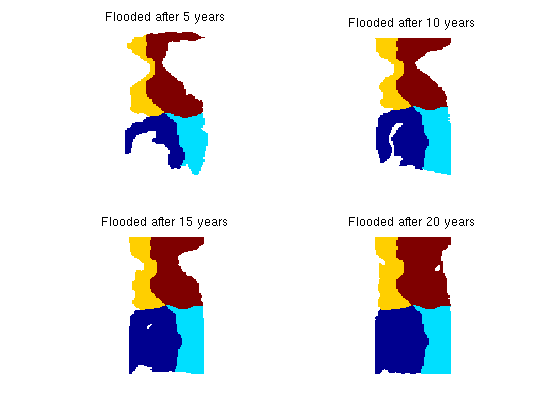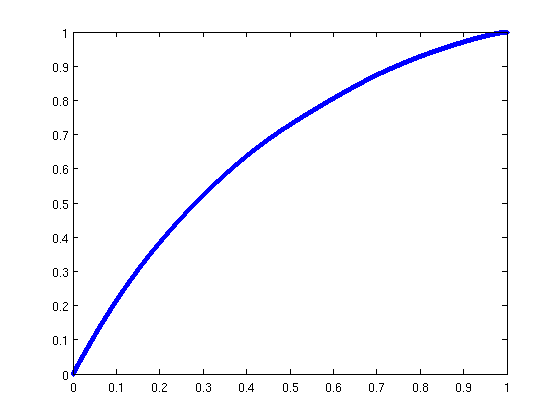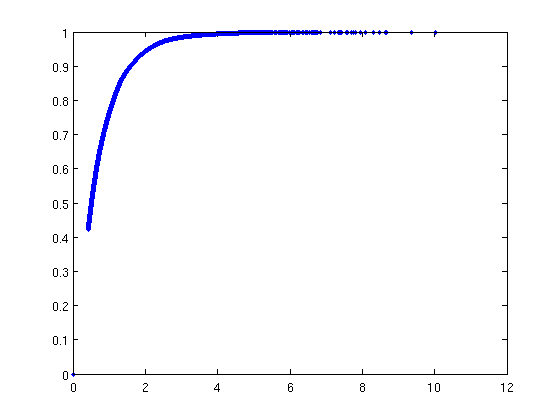#Basic flow diagnostics

In this example we will demonstrate how we can use time-of-flight and stationary tracer distribution computed using a standard finite-volume method to derive three different measures for heterogeneity:

1. flow-capacity/storage-capacity diagram,
2. the Lorenz coefficient
3. sweep efficiency

Technical description: see Shavali et al. (SPE 146446), Shook and Mitchell (SPE 124625).

## Set up and solve flow problem

As our example, we consider a standard five spot with heterogeneity sampled from Model 2 of the 10th SPE Comparative Solution Project.

```mrstModule add spe10
[G, W, rock] = SPE10_setup(25);
rock.poro = max(rock.poro, 1e-4);
fluid = initSingleFluid('mu', 1*centi*poise, 'rho', 1014*kilogram/meter^3);
rS = initState(G, W, 0);
S  = computeMimeticIP(G, rock);
rS = solveIncompFlow(rS, G, S, fluid, 'wells', W);
```

## Compute and display time-of-flight and tracer partitioning

First we compute time-of-flight which is the travel time from an injector to a given point in the reservoir, and stationary distribution of tracers injected continuously from each injetion well. From the latter, we can easily compute the volume flooded by each injector. Reversing the velocity field, we can cmopute the reverse time-of-flight (the travel time from an arbitrary point to the nearest producer) and the drainage volumes of each producer.

```D = computeTOFandTracer(rS, G, rock, 'wells', W);
subplot(1,2,1); plotCellData(G,D.ppart); axis equal tight
subplot(1,2,2); plotCellData(G,log10(sum(D.tof,2))); axis equal tight
```Threshold the tracer regions using time-of-flight to show the development of flooded regions

```for i=1:4
subplot(2,2,i); plotCellData(G,D.ppart, D.tof(:,1)<5*i*year);
title(['Flooded after ' num2str(i*5) ' years']); axis equal tight off;
end
```## Compute and display flow-capacity/storage-capacity diagram

Making an analogue to 1D displacement theory, the F-Phi curve is the equivalent to a plot of the fractional flow versus saturation.

```[F,Phi] = computeFandPhi(G.cells.volumes,D.tof);
figure, plot(Phi,F,'.');
```## Compute the Lorenz coefficient

The Lorenz coefficient is a popular measure of heterogeneity. It is equal to twice the area under the curve and above the F=Phi line. It varies between 0 (homogeneous displacement) to 1 (infinitely heterogeneous displacement).

```fprintf(1, 'Lorenz coefficient: %f\n', computeLorenz(F,Phi));
```
```Lorenz coefficient: 0.317024
```

## Compute and display sweep efficiency versus dimensionless time

Analogue: 1D displacement using the F-Phi curve as a flux function

```[Ev,tD] = computeSweep(F,Phi);
figure, plot(tD,Ev,'.');
```Published December 20, 2012